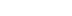/
article

# Building Your Own Subdivision Surfaces

Subdivision surfaces have received a lot of attention from both academics and industry professionals, people in the movie industry even apply subdivision techniques to create complex characters and produce highly detailed, smooth animation. Aaron Lee examines the process of converting triangular meshes (one of the most popular data representations) into subdivision surfaces.

Everyone who loves Quake 3 is impressed by the high quality graphics, light maps, and character animations. Although they have done an excellent job in painting the textual details, most of their characters consist of only several hundred triangles which cannot capture highly detailed geometry. In recent years, subdivision surfaces have received a lot of attention from both academics and industry professionals, people in the movie industry even apply subdivision techniques to create complex characters and produce highly detailed, smooth animation. This article examines how to convert triangular meshes (which is one of the most popular data representations) into subdivision surfaces.

What are Subdivision Surfaces?

The idea of subdivision surfaces was first introduced by Catmull & Clark and Doo & Sabin in 1978. Unlike traditional spline surfaces, subdivision surfaces are defined algorithmically. Recently there has been a lot of activity in the computer graphics research community and significant advances have been made in rendering, texture mapping, animation and compression of subdivision surfaces. They were also used in the production of Geri's Game and A Bug's Life. Geri's hands, head, jacket, and pants were each modeled using a single subdivision surface. The faces and the hands of Flick and Hopper were also modeled with subdivision surfaces. Momentum is building in the computer assisted geometric design (CAGD) to make subdivision surfaces one of the modeling primitives.

Why Subdivision Surfaces?

Subdivision surfaces lie somewhere in between polygon meshes and patch surfaces, and offer some of the best attributes of each. The well defined surface normal allows them to be rendered smoothly without the faceted look of low polygon count polygonal geometry, and they can represent smooth surfaces with arbitrary topology (with holes or boundaries) without the restriction in patches where the number of columns and rows has to be identical before two patches can be merged. Secondly, subdivision surfaces are constructed easily through recursive splitting and averaging: splitting involves creating four new faces by removing one old face, averaging involves taking a weighted average of neighboring vertices for the new vertices. Because the basic operations are so simple, they are very easy to implement and efficient to execute. Also because of the recursive nature, subdivision naturally accommodates level-of-details control through adaptive subdivision. This allows triangle budgets to be spent in regions where more details are needed by subdividing further.

What are the challenges?

The simple splitting process usually starts from a coarse control mesh, iterating this process several times will produce so-called semi-regular meshes. A vertex is regular if it has six neighbors (in triangle mesh) or four neighbors (in quadrilateral mesh). Vertices which are not regular are called extraordinary. Meshes that are coming from standard modeling packages or 3D scanning devices usually do no have a regular structure, hence there is a need to convert these irregular meshes into semi-regular meshes, a process known as remeshing. This article presents an algorithm that maps the original mesh onto a simple control mesh - base domain, thus deriving the parameterization for the original mesh. Having this mapping information (parameterization) allows us to perform the remeshing efficiently by subdividing the base domain and perturbing the new vertices to approximate the original geometry. From a signal processing point of view, we can treat it as a geometry resampling process. The beauty of this algorithm is that it is very simple and easy to implement.

Preparation Before Programming

Before explaining the algorithms, lets take a look at input. Input can be any arbitrary triangulated manifold mesh. By arbitrary we mean the mesh can have holes or boundaries, triangulated means the surface is approximated/discretized by a list of triangles. Manifold means it does not contain topological inconsistencies. Topological inconsistencies include more than two triangles sharing an edge or more than two corners meeting at one vertex. Make sure you have good, clean geometries.

Overview of Algorithm

The conversion algorithm contains two major steps. The first step is called mesh simplification. Mesh simplification has been well known to the game developers for creating levesl-of-detail or catering to different bandwidth and computational requirements. Most of the time, there is trade-off between model complexity and interactivity - i.e. a higher frame rate requirement usually is translated into simpler/coarser model. In this algorithm, mesh simplification is used only as a tool to derive a base domain so that resampling/remeshing can be acomplished. Developers are free to choose their favorite mesh simplification algorithm, I recommend Michael Garland's error quadrics simplification algorithm because it is open source and simple to understand. The second step is called remeshing; the goal is to create a subdivision connectivity (semi-regular) mesh with geometry sampled from the original mesh. As was mentioned earlier, subdividing the control mesh does not add any extra information to the mesh but only increases the complexity of the model. Hence there is a need to use the mapping information from the first step so that we know how to perturb these vertices to approximate the original mesh. (Figure 1).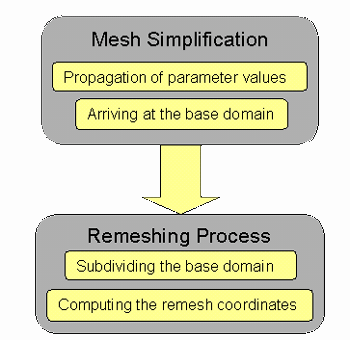Figure 1. The algorithm consists of two major steps. The first is mesh simplification, which allows us to derive the control mesh (base domain) and compute the mapping (parameterization of the original mesh) with respect to this base domain. The second step is geometry resampling (or remeshing). The goal is to obtain a subdivision connectivity mesh (through subdividing the base domain) and at the same time approximate the original mesh.

The 2D Case

Before diving into the 3D mapping algorithm, a look at the 2D curve case might be in order. The notion of subdivision connectivity does not make much sense here, but the conversion process can be treated as a regular sampling at the dyadic points. Simplification (removing alternating vertices in each step - as shown in Figure 2) is used to derive a simple line segment (base domain in red). Mid points are then inserted in the middle of each line segment by performing a linear interpolation between the two closest points (equivalent to the geometry resampling process) to complete the second phase (as show in Figure 3).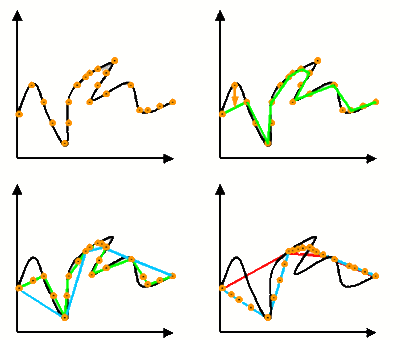Figure 2. This figure shows the result of applying the first phase of the algorithm. The idea is to do a simplification of the curve by removing every other vertex. At each simplification step, the removed vertex is mapped onto the simplified curve governed by the arc-length ratio. The end result would be a curve which maps onto a simple base line segment.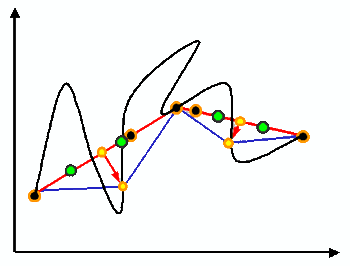Figure 3. This shows the second phase of the conversion algorithm in 2D. The yellow circles are the mid-points (analogy to the mid-points in the triangle subdivision case) of the base domain line segments. Their coordinates are computed from the linear interpolation of the two adjacent green vertices.

The 2D curve resampling process is computed as follows:

Insert a midpoint (yellow circle - dyadic point) on the red line segment (in Figure 3) and find out the two closest points (green circles) that were mapped from the original curve onto the red line segment. Then compute the ratio of the yellow circle within the two green circles. Based on the original geometry of the green circles and this ratio, we can linearly interpolate the coordinates and obtain the resample geometry of this yellow circle on the original curve.

Now with this simple idea, let's extend it to 3D.

To illustrate the algorithm, I'm using Mike Garland's excellent simplification algorithms from . The software can be downloaded from his site at http://graphics.cs.uiuc.edu/~garland
/software/qslim.html
. The nice thing about this simplification is that it is very fast and easy. It can simplify highly detailed polygonal surface models automatically into faithful approximations containing much fewer polygons. The core of the algorithm employs an error metric called Quadric error metric to provide a characterization of local surface shape. This metric is both fast to evaluate and does not take up too much memory space. The details of the algorithm can be found at http://graphics.cs.uiuc.edu/~garland/research/thesis.html.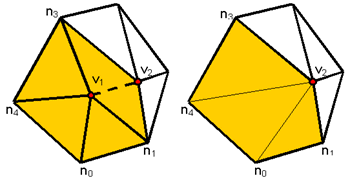Figure 4. The contraction of edge v1v2 will remove vertex v1 and it's adjacent triangles (old triangles), thus creating a hole and retriangulation is performed (new triangles are formed).

Propagating mapping information

Each simplification step collapses an edge, removing one vertex and deleting the triangles that surround the vertex and retriangulating the hole. In Figure 4, edge v1v2 is collapsed and v1 is removed. In order to preserve some form of mapping between adjacent levels, we are going to compute a 4-tuple (a, b, g, T) that describes which new triangle T that v1 is going to associate with. The (a, b, g) tuple is the barycentric coordinates of v1 within the triangle T. To perform this association for each simplification step, flatten the 1-ring (as shown in Figure 5) of v1 onto a 2D plane. This planar flattening is achieved by computing the za map. It involves scaling the angle sum subtend at v1 to 2p or p in the boundary case and raise the length of each edge subtend at v1 to power a.

The 4-tuple can be calculated as in Figure 5: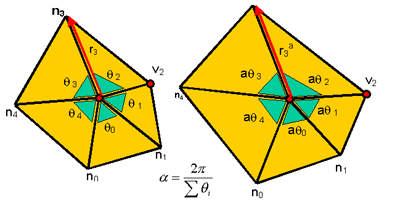Figure 5. To compute the 4-tuple, first flatten the 3D umbrella (1-ring) around v1 onto a 2D plane. The scaling factor a is used to ensure the angle sum is 2p, notice also that the length is scaled by the power a. Once the umbrella is flattened, compute the location of v1 in the new triangulation.

Code Snippets

RemoveVertex( Vertex V ) {
PlanarFlatten( V );
Valid = TryRetriangulate( V );
if ( Valid ) {
AssignParameter( V );
Reparameterize( V );
DoTriangulate( V );
};
};

The basic skeleton of the vertex removal is very simple. First perform a planar flattening to flatten the 3D 1-ring neighbors of vertex V (the umbrella) onto a 2D plane according to Figure 5 description, i.e. scaling the edge length and the angles subtended at vertex V. After flattening the umbrella use a retriangulation routine (e.g. a Delaunay triangulation) to remove the old triangles and try inserting new triangles. Make sure that the new triangles do not overlap with the existing triangles in the mesh, otherwise it will create topological inconsistencies and the mesh will not be a manifold (i.e. no more 2 triangles share an edge). If the new configuration is valid, proceed to compute the 4-tuple parameter for vertex V. The computation consists of two steps. The first step is to assign a new 4-tuple parameter for vertex V which involves the calculation of the barycentric coordinates and the associating triangle as indicated in Figure 6. The second step is to update the parameter values of those vertices which are currently associating with the old triangles. The function CalcNewParameters( Vi ) will perform the corresponding update according to Figure 7.

AssignParameter( Vertex V) {
Tuple = CalcBaryCoord( V );
InsertTuple( V, Tuple );
};
Reparameterize( Vertex V ) {
ForEachFaceAroundVertex( V , Fi ) {
ForEachAssociatedVertex( Fi , Vi ) {
NewTuple = CalcNewParameters( Vi );
UpdateTuple( Vi , NewTuple );
};
};
};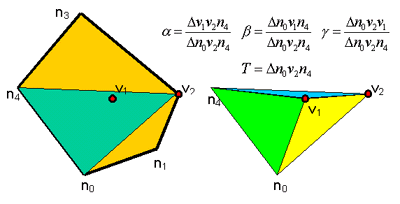Figure 6. In this figure, v1 is mapped onto the green triangle, proceed to compute the barycentric coordinates (a, b, g) and the new triangle that v1 will be associated with.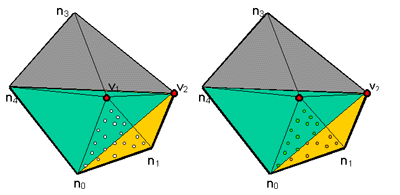Figure 7. When removing the 1-ring triangles, be sure to update the parameter values of those vertices which were previous associated to the new triangulation. This example shows the reparameterization of the white vertices onto the new triangulation.

If there are vertices which were associated with the old triangles, we also need to update their parameters due to the retriangulation (old triangles will be destroyed). The update can be computed in way similar to the previous step.

At the end of the simplification there will be a simple control mesh, called a base domain. For each vertex that was removed in the simplification hierarchy, it will have the 4-tuple indicating which base domain triangle it is associating with and its barycentric coordinates. This complete the first phase of the algorithm.

Examples of running the algorithm

The following images show the results of performing the first phase of the algorithm on a 3-holes torus. Although this model is a bit simple, it does show the general ability of the algorithm to handle a genus-3 object (containing 3 handles).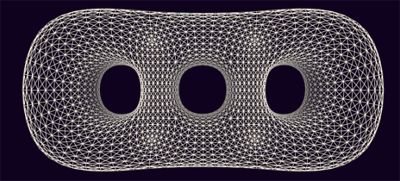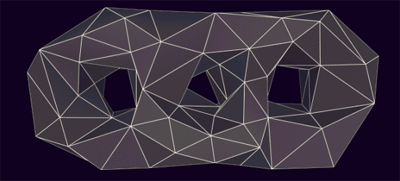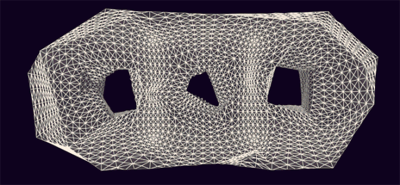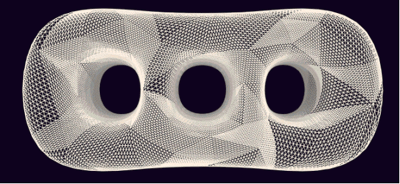The first image shows the original triangular mesh, while the second image shows the base domain arrived at from the simplification routine. The third image demonstrates the visualization of mapping each vertex onto the base domain - thus shrink-wrapping the original mesh onto the base domain with each vertex having a 4-tuple association. The fourth image shows the result of subdividing the base domain and perturbing the new vertices to closely approximate the original mesh.

At this point, the mapping computation is finished. One way to look at the mapping is consider that each vertex has a 4-tuple parameter which tells which base domain triangle it is associating with and it's location (given by the barycentric coordinates) within this base domain triangle. Another way to visualize the mapping is to imagine collapsing/wrapping the original mesh triangulation (treated as a graph) on top of the base domain.

Control mesh subdivision

To create a mesh with subdivision connectivity, simply subdivide the control mesh a few times by splitting an old triangle into four new triangles. The new edge vertices are called dyadic points. Notice that this 1-4 split produces vertices with 6 neighbors. All of the new vertices introduced are regular. The most common subdivision schemes are Loop subdivision and Catmull-Clark subdivision. Loop subdivision is a scheme for triangular meshes while Catmull-Clark subdivision is for quadrilateral meshes. This article demonstrates the Loop subdivision method, as it's a natural choice for triangle meshes. The limit surface of the subdivision will be a smooth surface with C2 continuity everywhere except at the extraordinary vertices. The next step is to perturb the vertices in such a way that the subdivision mesh can approximate the original mesh. This is what is called the geometry resampling.

Perturbing vertex coordinates

Before examining how to move these subdivision vertices to approximate our original mesh geometry, there is a need to fix on some notations and introduce the edge terminology so that the algorithims can be explained. Basically, an edge in the mesh is represented as a directional edge containing pointers to its origin vertex (e.Org), destination vertex (e.Dest), previous edge from its destination vertex (e.Dprev) and next edge from its origin vertex (e.Onext), as show in Figure 8.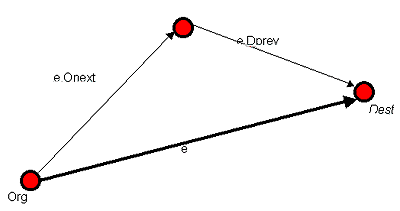Figure 8. Directional edge notation and its pointers to neighboring data structures.

Once we define the edge algebra we can proceed to the next step: perturbing the vertex coordinates.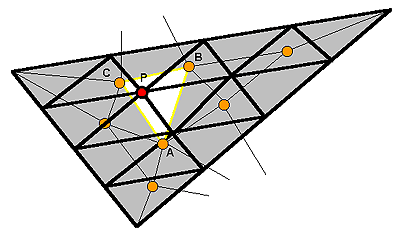Code Snippets

To compute the vertex coordinates for the subdivision vertices, first find the triangle in the flatten mesh on the base domain which contains the dyadic points (similar to the 2D case in Figure 3 where we locate the two green circles). The triangle location problem is reduced to a point location problem. M denotes the flattened original mesh on the base domain, and V is the red dyadic vertex. The white triangle containing V can be located using the routine in listing 1.

Once the triangle in the collapsed original mesh containing the dyadic points is found a linear interpolation can be used to find the resample location: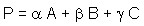Where P is the dyadic point, Triangle ABC is the collapsed original triangle with original geometry. (a, b, g) is the barycentric coordinates of P within the triangle ABC in the collapsed graph. Hence P will be a resample point on the piecewise linear original mesh geometry. Repeating this process for all new vertices allows the perturbing of the subdivision connectivity mesh to approximate the original geometry. The following examples show the results.

Horse and Venus Results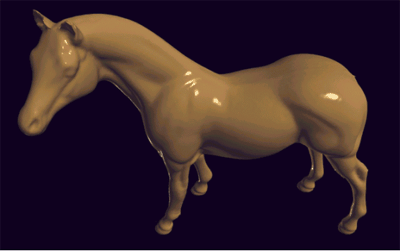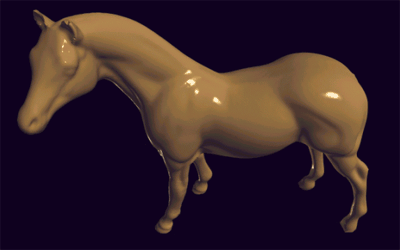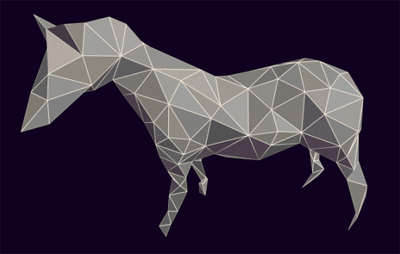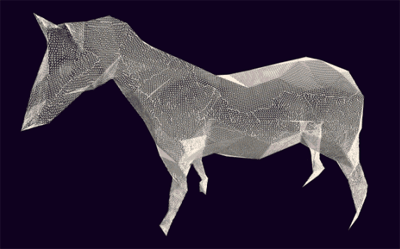Figure 9. The first picture shows the original horse model with irregular connectivity. The second picture shows the result of geometry resampling, the third picture illustrates the base domain (as a result of mesh simplification) while the fourth picture demonstrates the parameterization of the original mesh with respect to the base domain.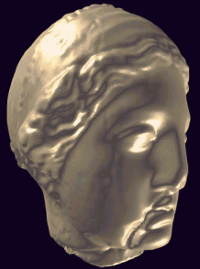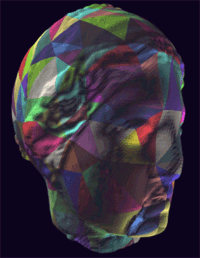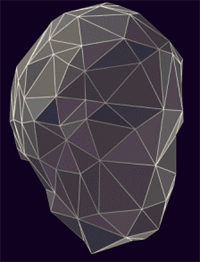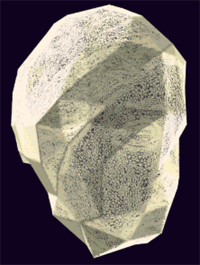Figure 10. Another example (the Venus head) of running our algorithm. The second pictures shows the subdivision connectivity patch structure (color patches) with the base domain and visualization of the parameterization.

To sum up, this article presents a simple algorithm to convert triangular meshes into subdivision surfaces. The algorithm is both easy to understand and implement, the main idea being to recast the problem as geometry resampling and compute the mapping (parameterization of the original mesh) with respect to a simple base domain. Subdivision surfaces open the doors of opportunity to character animation, free form surface design and efficient rendering. The next part of this article looks at applying subdivision surfaces techniques to these tasks. Stay tuned!

### Latest Jobs

#### Treyarch

Playa Vista, California
6.20.22
Audio Engineer

#### Digital Extremes

6.20.22
Communications Director

6.20.22
Senior Producer

#### Build a Rocket Boy Games

Edinburgh, Scotland
6.20.22
More Jobs